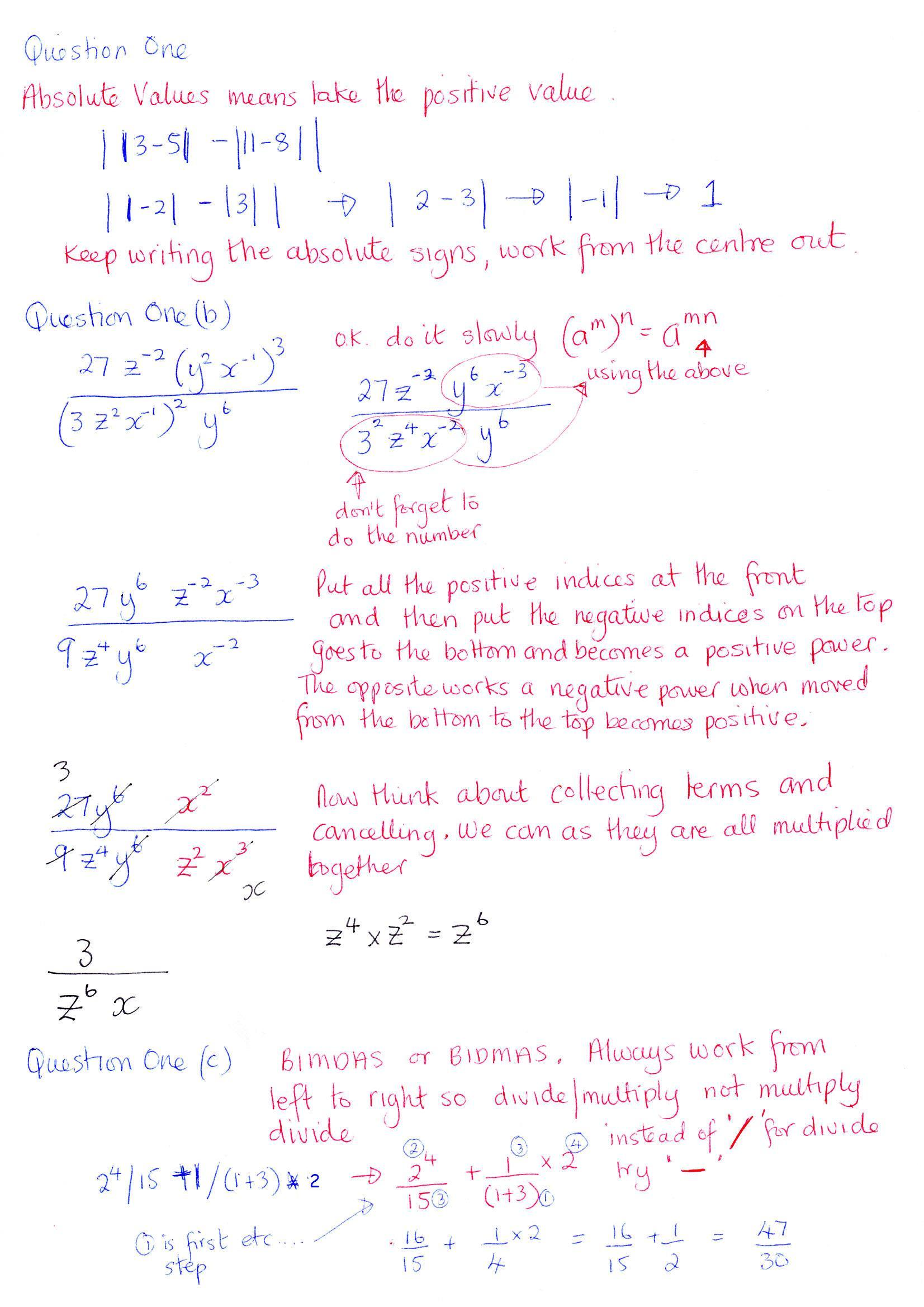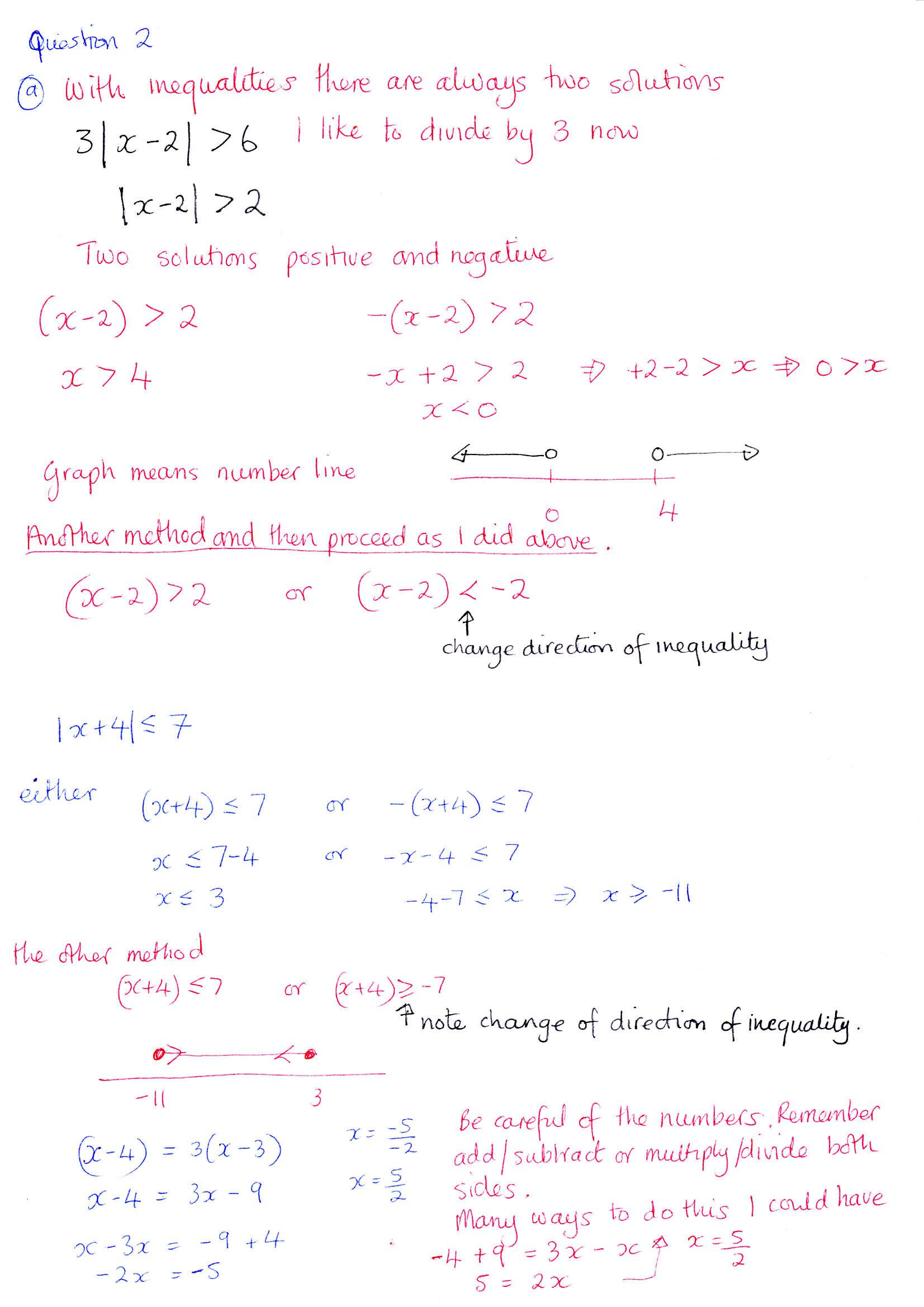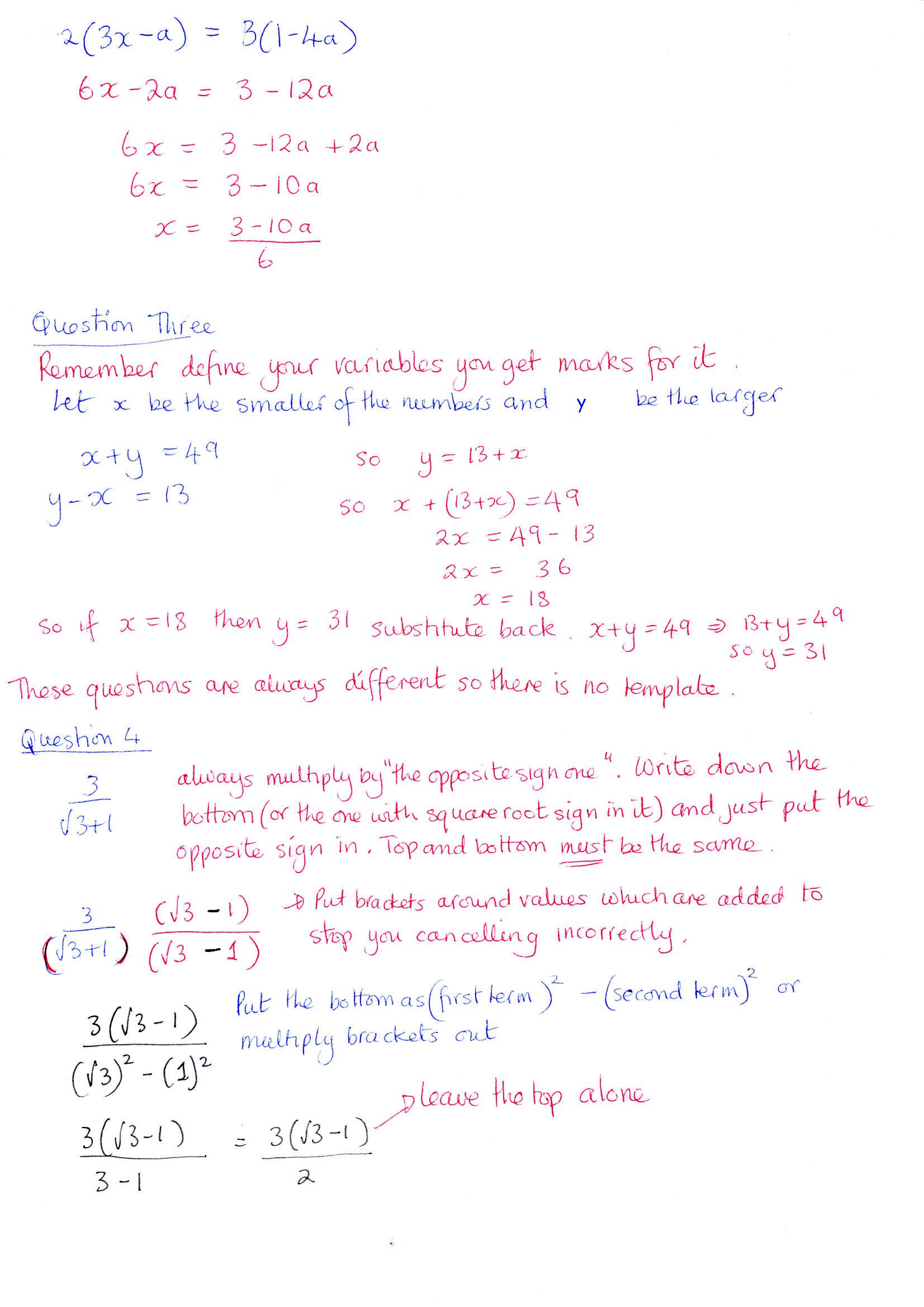Another way of thinkingDivide the expression by where the plus or minus signs occur outside the brackets and then combine these answers at the end  2 4  / 15  +   1 / (1+3)*2Work out the red and stop as you have reached a plus sign 16/15.  Now 1/4*2   do this in the order you say it, so one divided by four is  a quarter multiplied by 2 is 1/2                              16/15 + 1 / 2 = 47 / 30